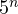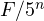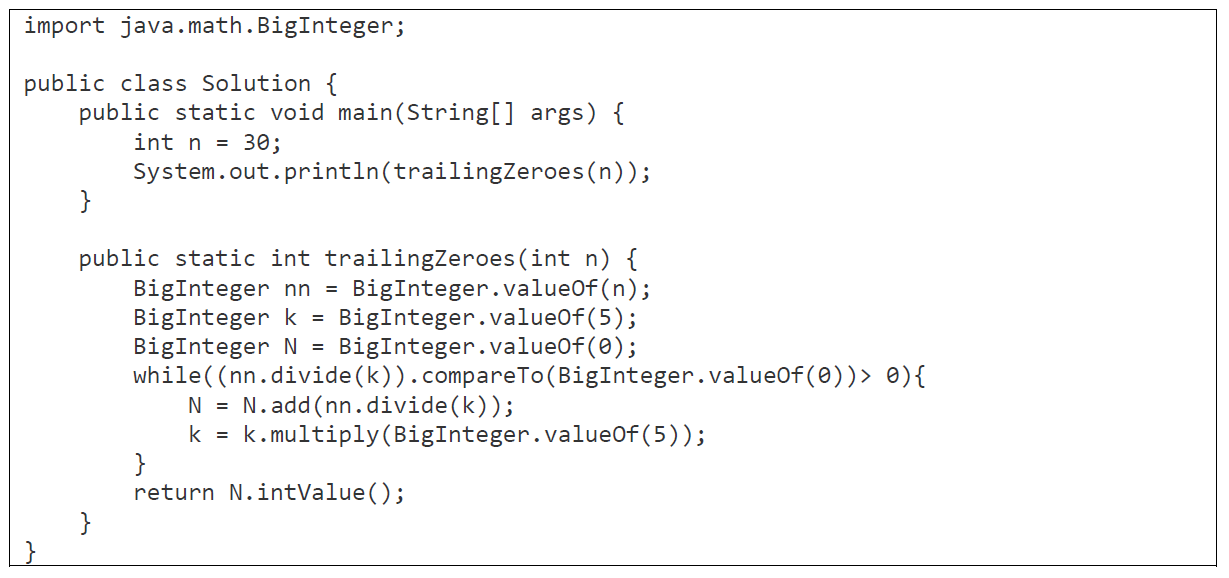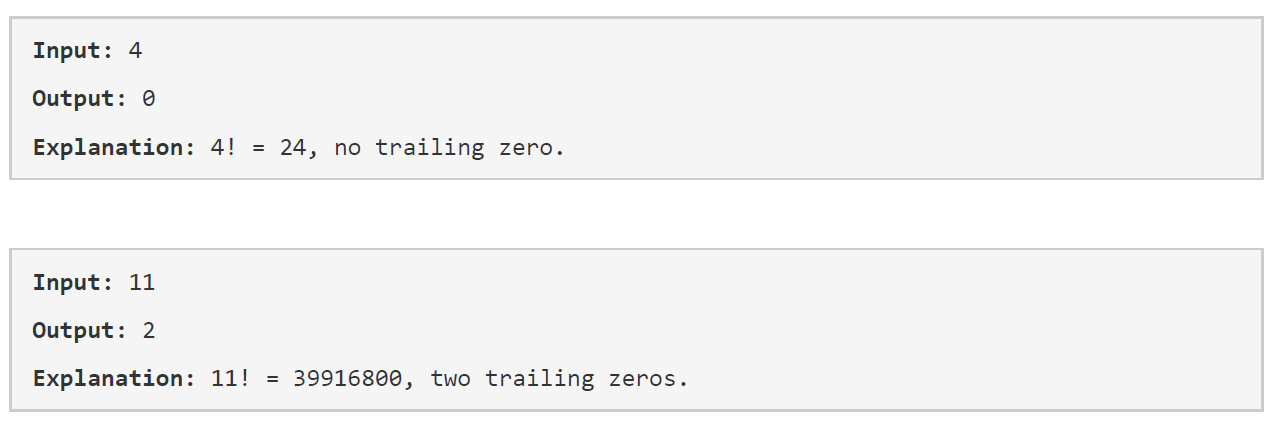# Factorial Trailing Zeroes: Sample

Write a Java program that returns the number of trailing zeroes for a given n! factorial.

Solution:

Calculation of the factorial takes a lot of time. Thus, a dependency was found that the factorial number has a number of trailing zeros proportional to, where n is the number of trailing zeros. Thus, we need to add the number of times when the factorial number can be divided by.

Number =The algorithm is shown below: